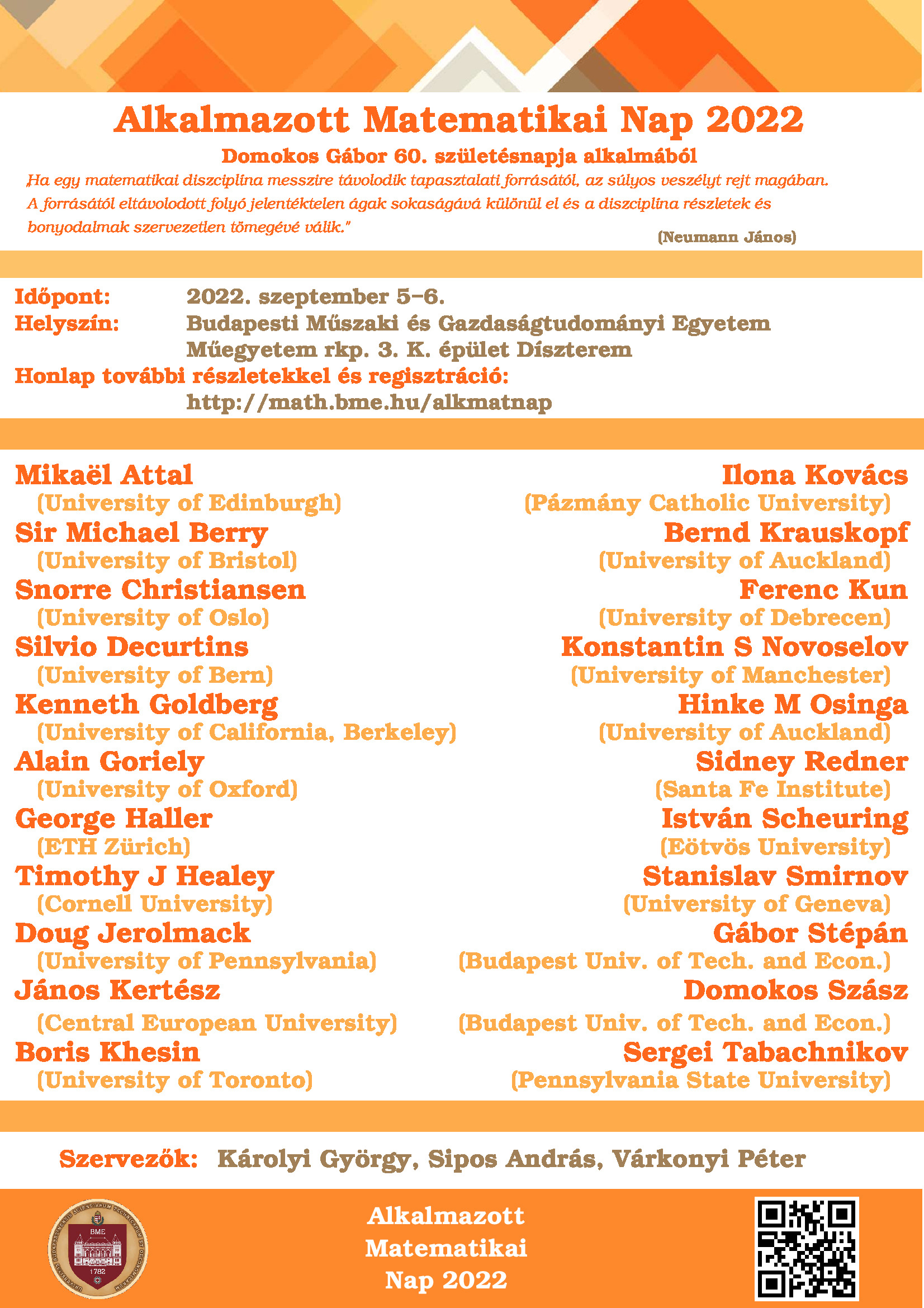## Applied Mathematics Without Borders

A series of lectures were presented at BME Applied Mathematics Day – Applied Mathematics Without Borders 2022, organized on 5-6 September, BME central building, 1st floor, “Díszterem” on the occasion of the 60th anniversary of Gábor Domokos.## Talk at the DDG2 conference

#### Mechanical complexity of complex polyhedra

Discrete Geometry Days 2, Budapest

Abstract: Let P be a convex polyhedron with faces, edges and vertices, and assume it is realized as a homogeneous solid having stable, saddle-type and  unstable equilibrium configurations (i.e., standing on a face, edge or vertex over a horizontal surface). Let mechanical complexity C(Pof be defined as the difference between the sums f + e + v and  S + H + and define mechanical complexity C(S,Uof primary equilibrium classes (S,U)^as the minimum of mechanical complexities of all polyhedra having stable and unstable equilibria. In this talk we show that mechanical complexity in any non-monostatic (i.e., and 1) primary equilibrium class (S,U)^equals the minimum of 2(f+vSUover all polyhedra in the same class having simultaneously faces and vertices; thus, mechanical complexity of a class (S,U)^is zero if and only if there exists such that f = and v = U. We also give both upper and lower bounds for C(S,Uin the cases S 1 and U = 1  (a problem related to a question of Conway and Guy in 1969 asking about the minimal number of faces of convex polyhedra with only one stable equilibrium point), and we offer a complexity-dependent prize for C(1,1). Joint work with Gábor Domokos, Zsolt Lángi, Krisztina Regős and Péter T. Varga.